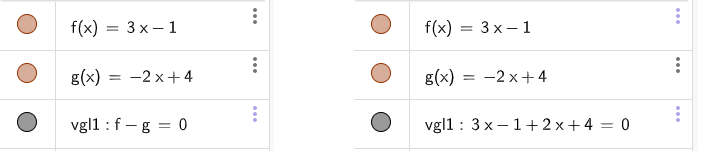# substraction in algebra

ccambre shared this problem 1 year ago
Solved

with inputs for f and g the equation f-g is wrongly calculated because strangely the +4 isn't changed into -4 in a basic substraction.1

typing f(x)-g(x)=0 is OK1

muchas gracias. Writing brackets indeed prevents the error.

Graphically f - g shows the line x = 1 while in the Algebra it gives x = -3/5.

The same solution in the Algebra as in the Graphics could be expected in any case.1

Fixed for v659